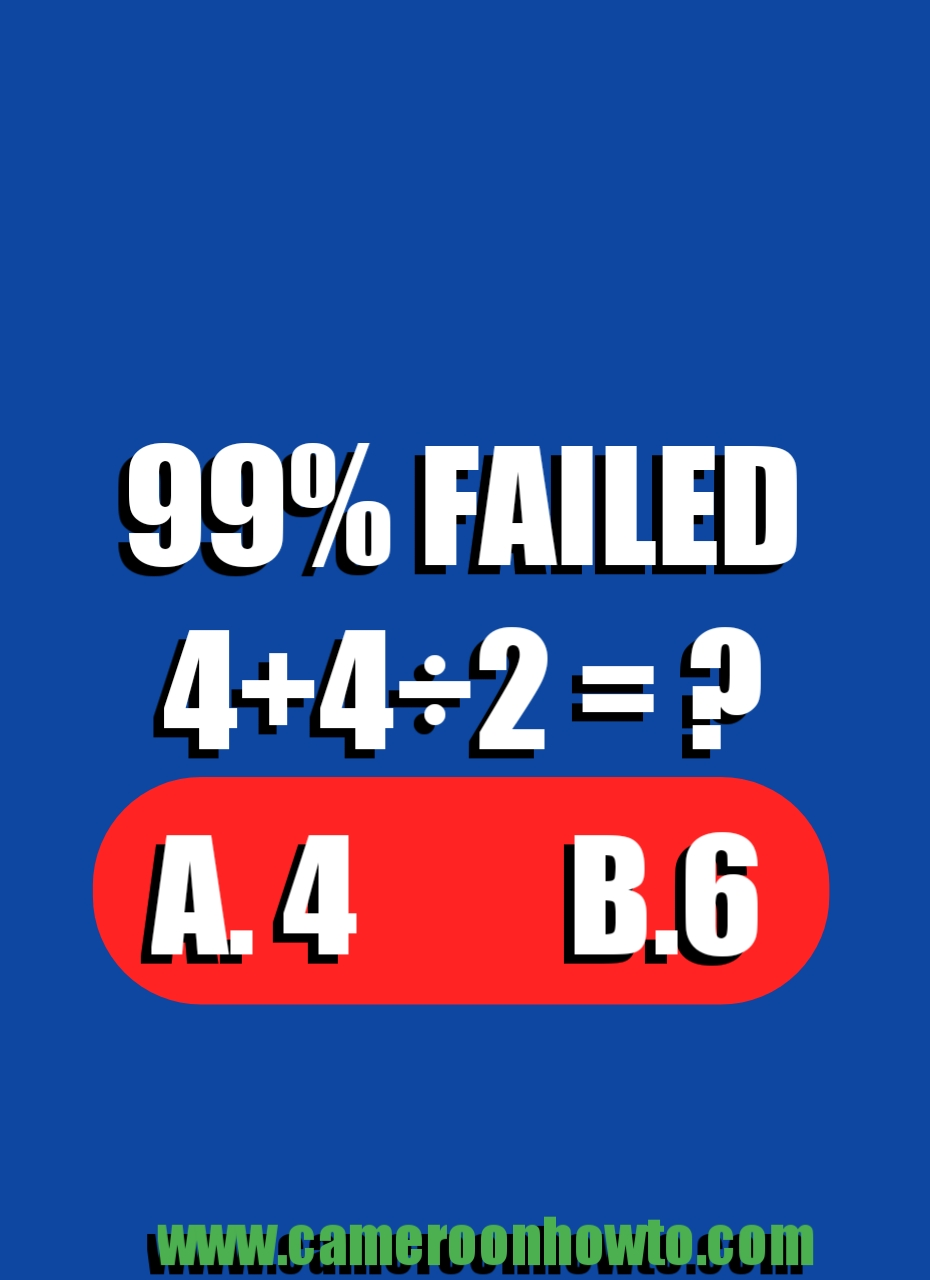0

BODMAS rules.What's 4+4÷2 =? answer

This math quiz " 4+4÷2=?"  and Similar ones sparked a massive debate online.

When it was published on Facebook, Many people failed it. Today I will solve it and give you the correct answer.

The correct answer to 4+4÷2 would be 6 not 4, this is because we need to apply BODMAS correctly when solving this kind of Quizzes. Check the full solution below.

Many people love solving math quiz puzzles and riddles to refresh their brains. Many people love sharing dare games and challenges to friends, family members and loved ones.

So, 4+4÷2=?

4÷2 = 2.

Implies 4 + 2 =6.

Therefore, the correct answer to 4+4×2 = 6.

Conclusion

If you are using a Calculator, after inputting all the numbers, press equal to then the answer will be 6.

If you input 4+4= 8, 8÷2 = 4. This answer is correct but is against BODMAS rules.

Tags